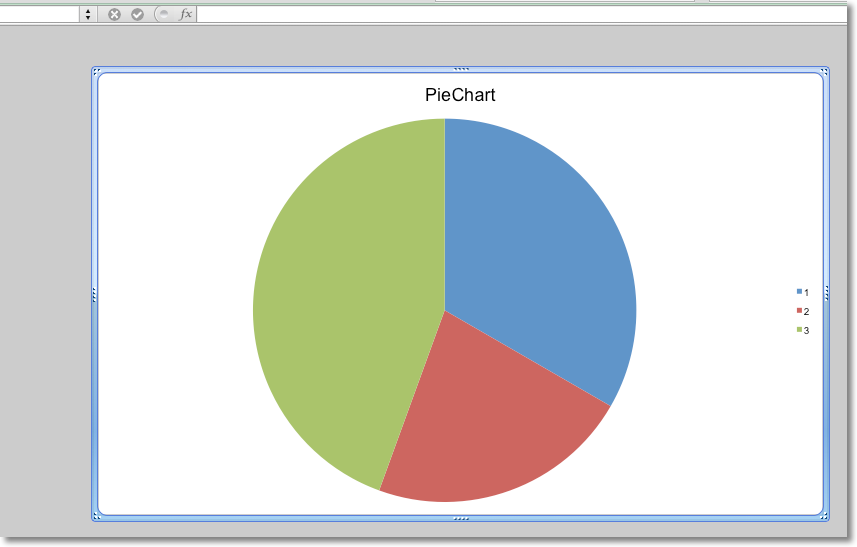# Chartsheets¶

Chartsheets are special worksheets which only contain charts. All the data for the chart must be on a different worksheet.

```from openpyxl import Workbook

from openpyxl.chart import PieChart, Reference, Series

wb = Workbook()
ws = wb.active
cs = wb.create_chartsheet()

rows = [
["Bob", 3],
["Harry", 2],
["James", 4],
]

for row in rows:
ws.append(row)

chart = PieChart()
labels = Reference(ws, min_col=1, min_row=1, max_row=3)
data = Reference(ws, min_col=2, min_row=1, max_row=3)
chart.series = (Series(data),)
chart.title = "PieChart"